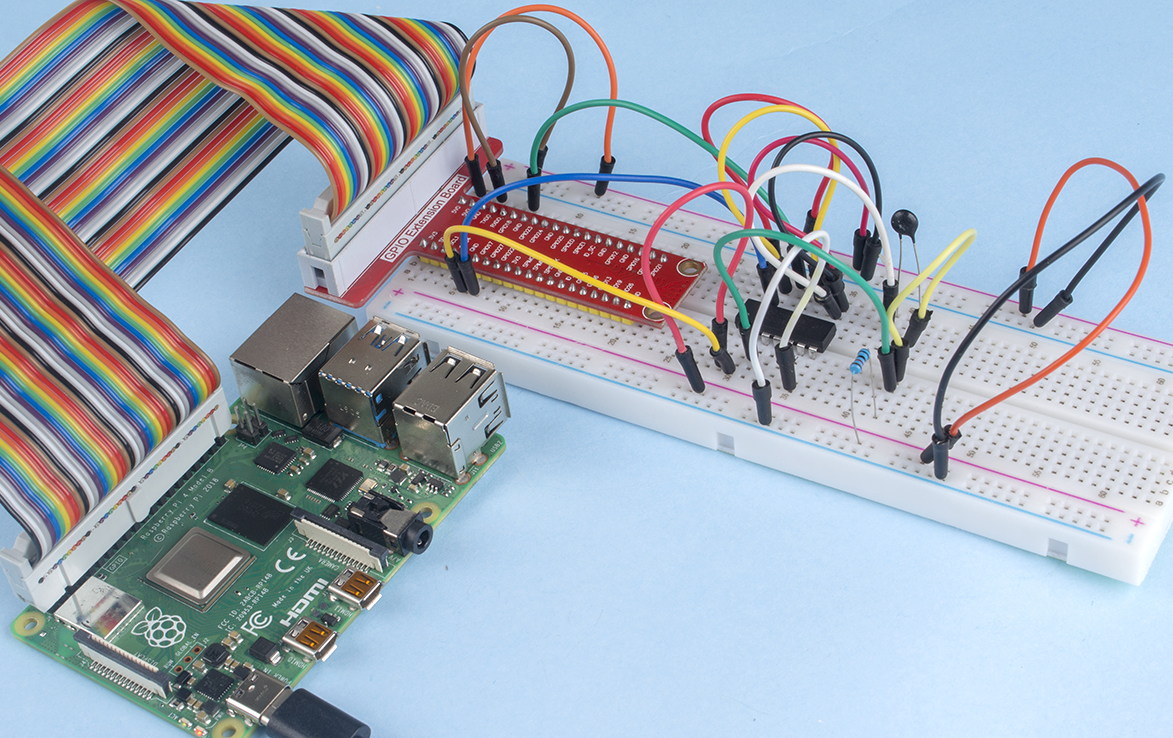# 2.2.2 Thermistor¶

## Introduction¶

Just like photoresistor can sense light, thermistor is a temperature sensitive electronic device that can be used for realizing functions of temperature control, such as making a heat alarm.

## Required Components¶

In this project, we need the following components.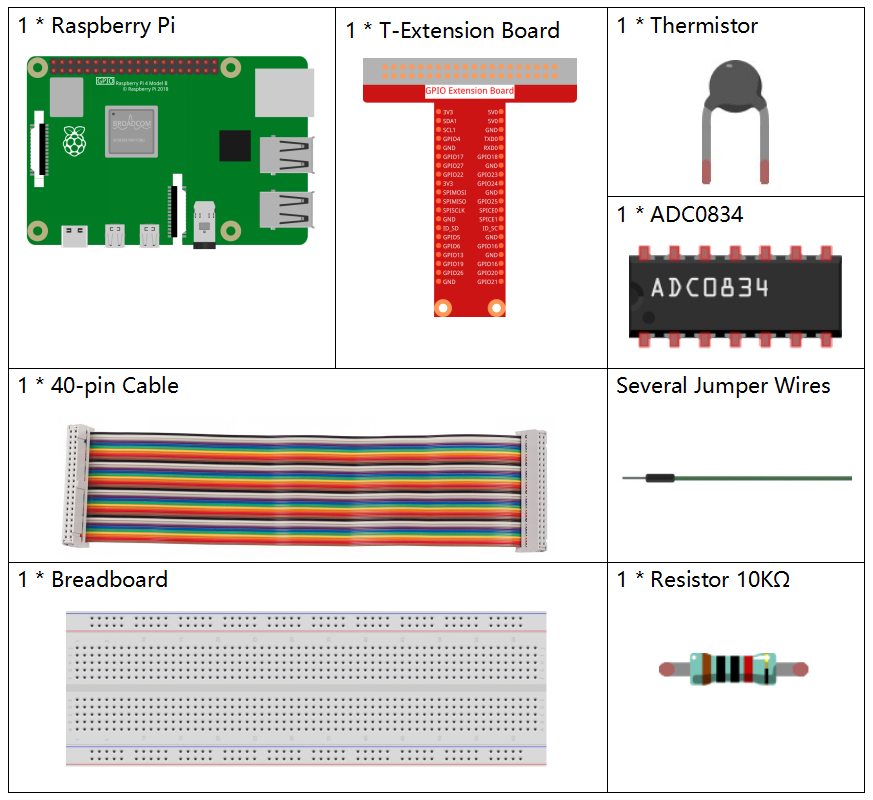Name

ITEMS IN THIS KIT

Raphael Kit

337

Raphael Kit

COMPONENT INTRODUCTION

GPIO Extension Board

Jumper Wires

Resistor

Thermistor

-

## Schematic Diagram¶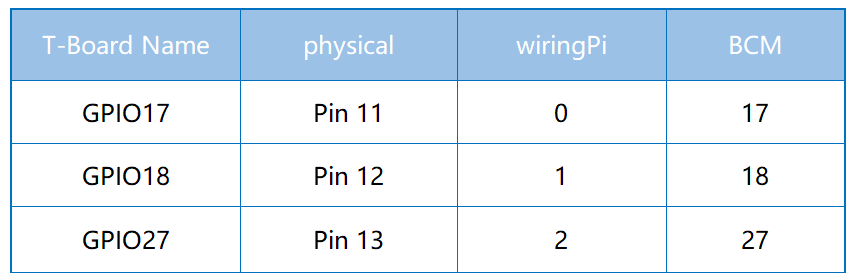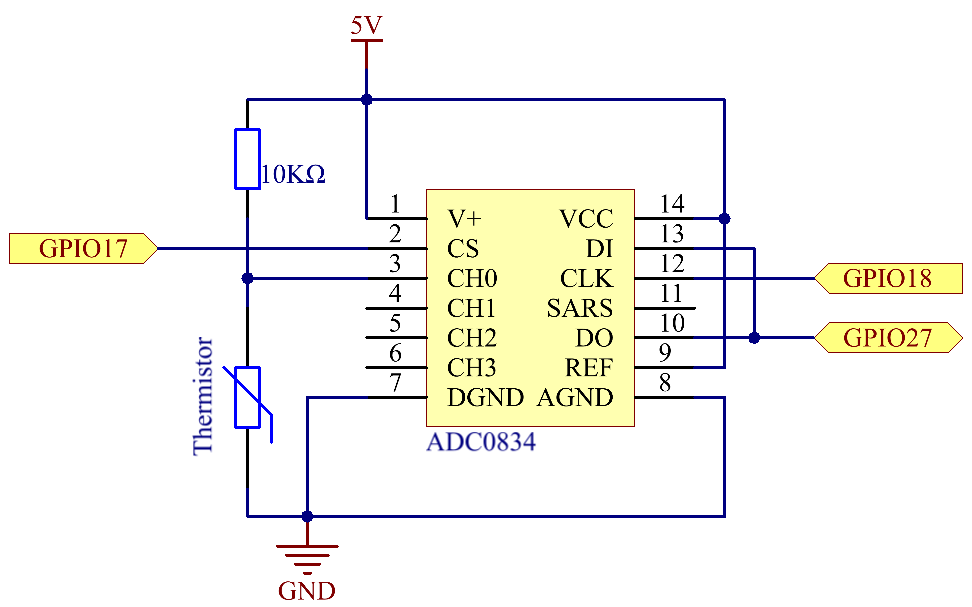## Experimental Procedures¶

Step 1: Build the circuit.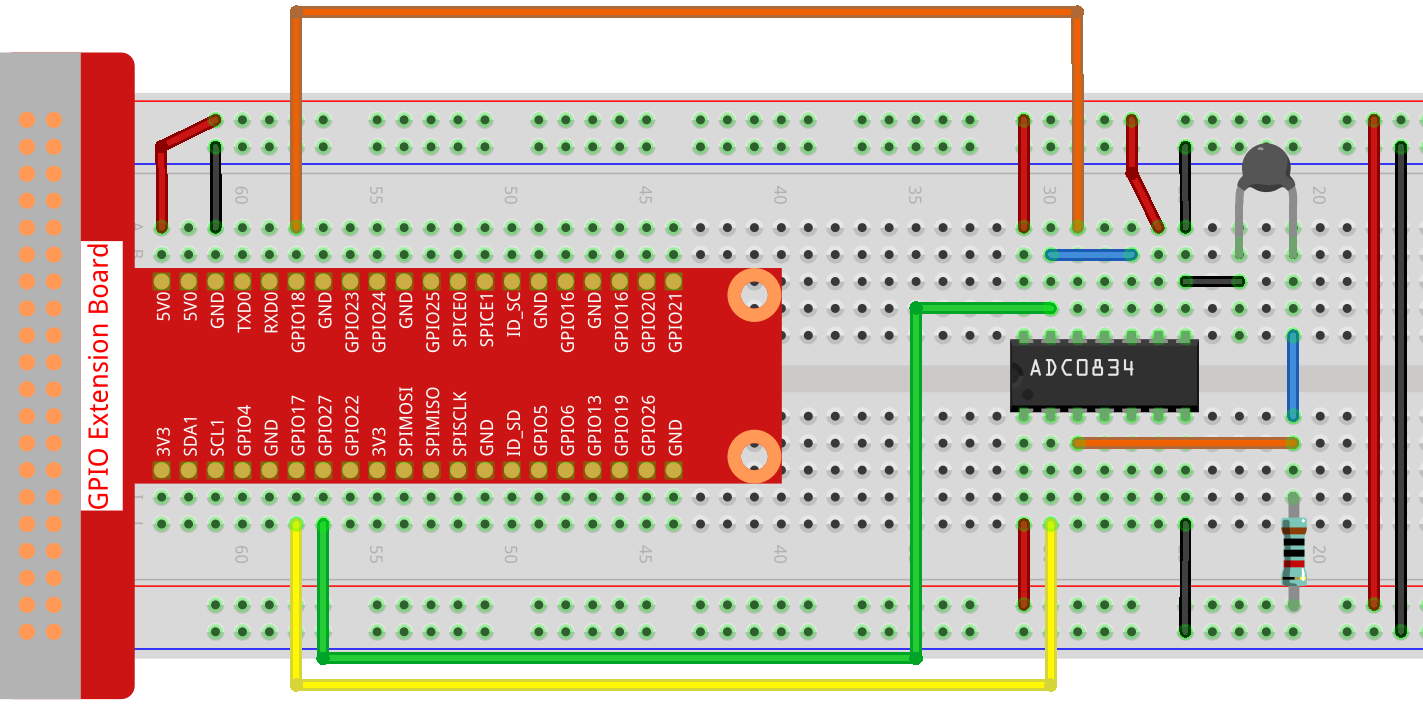Step 2: Go to the folder of the code.

```cd ~/raphael-kit/c/2.2.2/
```

Step 3: Compile the code.

```gcc 2.2.2_Thermistor.c -lwiringPi -lm
```

Note

-lm is to load the library math. Do not omit, or you will make an error.

Step 4: Run the executable file.

```sudo ./a.out
```

With the code run, the thermistor detects ambient temperature which will be printed on the screen once it finishes the program calculation.

Note

If it does not work after running, or there is an error prompt: "wiringPi.h: No such file or directory", please refer to Install and Check the WiringPi.

Code

```#include <wiringPi.h>
#include <stdio.h>
#include <math.h>

typedef unsigned char uchar;
typedef unsigned int uint;

{
uchar i;
uchar dat1=0, dat2=0;
int sel = channel > 1 & 1;
int odd = channel & 1;

// Start bit
//Single End mode
// ODD
//Select

for(i=0;i<8;i++)
{

}

for(i=0;i<8;i++)
{
}

return(dat1==dat2) ? dat1 : 0;
}

int main(void)
{
unsigned char analogVal;
double Vr, Rt, temp, cel, Fah;
if(wiringPiSetup() == -1){ //when initialize wiring failed,print messageto screen
printf("setup wiringPi failed !");
return 1;
}

while(1){
Vr = 5 * (double)(analogVal) / 255;
Rt = 10000 * (double)(Vr) / (5 - (double)(Vr));
temp = 1 / (((log(Rt/10000)) / 3950)+(1 / (273.15 + 25)));
cel = temp - 273.15;
Fah = cel * 1.8 +32;
printf("Celsius: %.2f C  Fahrenheit: %.2f F\n", cel, Fah);
delay(100);
}
return 0;
}
```

Code Explanation

```#include <math.h>
```

There is a C numerics library which declares a set of functions to compute common mathematical operations and transformations.

```analogVal = get_ADC_Result(0);
```

This function is used to read the value of the thermistor.

```Vr = 5 * (double)(analogVal) / 255;
Rt = 10000 * (double)(Vr) / (5 - (double)(Vr));
temp = 1 / (((log(Rt/10000)) / 3950)+(1 / (273.15 + 25)));
cel = temp - 273.15;
Fah = cel * 1.8 +32;
printf("Celsius: %.2f C  Fahrenheit: %.2f F\n", cel, Fah);
```

These calculations convert the thermistor values into Celsius values.

```Vr = 5 * (double)(analogVal) / 255;
Rt = 10000 * (double)(Vr) / (5 - (double)(Vr));
```

These two lines of codes are calculating the voltage distribution with the read value analog so as to get Rt (resistance of thermistor).

```temp = 1 / (((log(Rt/10000)) / 3950)+(1 / (273.15 + 25)));
```

This code refers to plugging Rt into the formula TK=1/(ln(RT/RN)/B+1/TN) to get Kelvin temperature.

```temp = temp - 273.15;
```

Convert Kelvin temperature into degree Celsius.

```Fah = cel * 1.8 +32;
```

Convert degree Celsius into Fahrenheit.

```printf("Celsius: %.2f C  Fahrenheit: %.2f F\n", cel, Fah);
```

Print centigrade degree, Fahrenheit degree and their units on the display.

## Phenomenon Picture¶